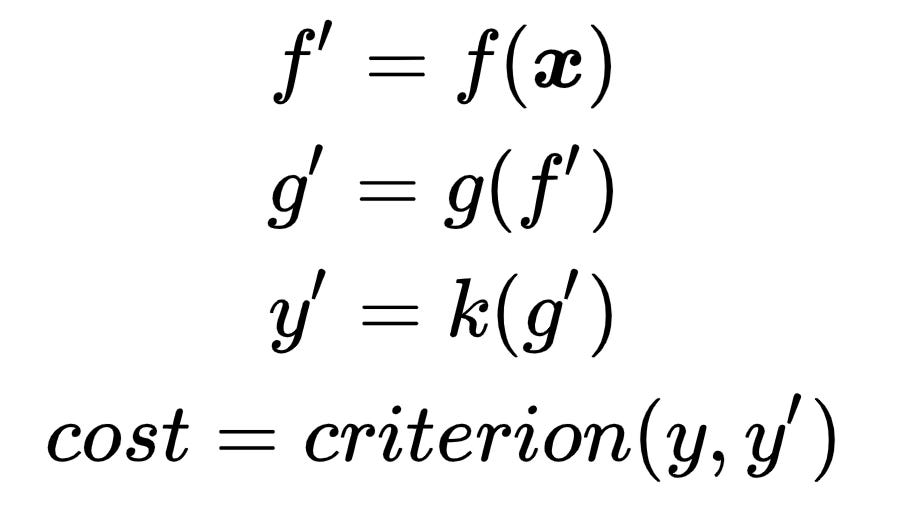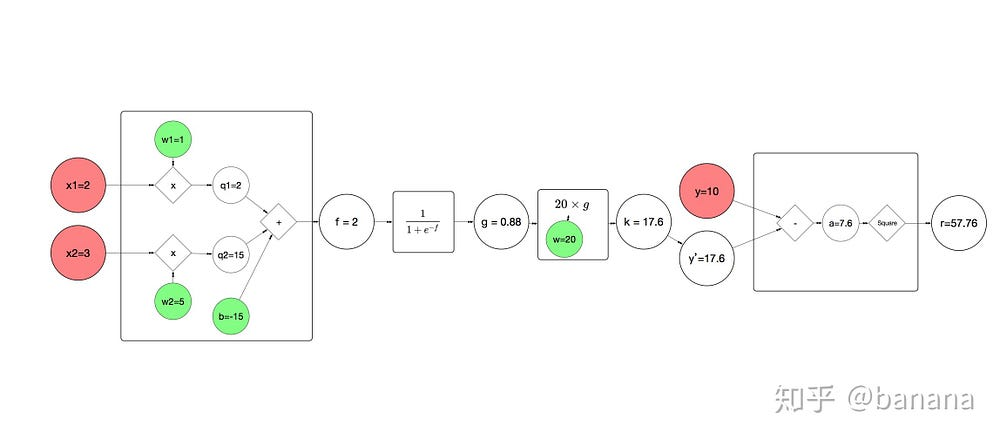# Deep learning and chain rule of calculus

Source: Deep Learning on Medium

## Foreword

In recent years, deep learning has swept through many fields of machine learning, computer vision, natural language processing, recommendation systems, etc., and has become the best method in these fields. One of the most important elements of deep learning or neural networks is the backpropagation algorithm. The backpropagation algorithm has a very close relationship with the derivative chain rule in mathematics. The current popular network structure, all follow this rule, such as LeNet, AlexNet, GoogLeNet, VGG, ResNet in computer vision. This rule has some implications in the previous article on the gradient descent and the derivation. It is specifically mentioned here again to show its working method more clearly.

#### The relationship between deep learning and chain rule of calculus

From the previous article gradient descent and the derivation, we know that for the map that can be derived, we can use the variable parameters of the map to deduct and use the gradient descent method to slowly make the variable parameters close to what we want which makes the cost smaller. Deep learning imposes multiple mappings and performs multiple mapping operations on the input. In theory, the performance of multiple mapping will be higher than a single mapping. After optimization, the cost can be made smaller. The derivation chain rule is a rule used to calculate cost derivate variable parameters in each map in a order.

#### The chain rule of calculus

Suppose cost is calculated as follows, the input is x and the target value is y,

If you want to calculate d(cost) / d(x), x can be a number, a vector, or a matrix. You can calculate d(f’) / d(x)d(g’) / d(f’)d(cost) / y’ to get d(cost) / d(x). In machine learning, the three functions here, f, g, k represent different mappings, and the criterion is also understood as a mapping, except that the input here adds the target value y. The x here represents the input data, but the meaning of the input value is not significant, because we can’t change the data to make our target cost smaller. The actual situation is to change the variable contained in each map. The variables are derived.

For example, if you use w𝚏 to represent the variable in function f, you can now calculate the derivative of cost to w𝚏. You can calculate it as follows. Before displaying the calculation method, rewrite the previous expression here. Include w𝚏,

In order to calculate d(cost) / d(w𝚏), we can get it by calculating

This is from the chain rule of calculus.

For another example, if w𝚐 is used to represent the variable in function g, now we need to calculate the derivative of cost for w𝚐, which can be calculated as follows. Before displaying the calculation, the expression is rewritten based on the previous example to include w𝚐.

In order to calculate d(cost) / d(w𝚐), we can get it by calculating

From the previous examples, f, g, and k represent different mappings. Each mapping only needs to be responsible for itself. It only needs to calculate the derivative of its output to the input, such as d(g’)/d(f’), d(y’)/d(g’), d(cost)/y’ which used in the above two examples. and what is the derivative of some variable output to itself, such as d(g’)/d(w𝚐), d(f’)/d(w𝚏)

### An example of TorchEach block is a map, the first three blocks are the three mappings from input to output. The last block is the difference between the evaluation output and the target value, used to evaluate the current input to output mapping.

Each block is a map, the first three blocks are the three mappings from input to output. The last block is the difference between the evaluation output and the target value

1. The input here is a two-dimensional vector, x = [x₁, x₂] = [2, 3]
2. The first mapping: f’=f(x) = wx₁ + wx₂ + b = 1 ⨉ 2 + 5 ⨉ 3 + -15 = 2 , can be represented by nn.Linear in the Torch code.
``require 'nn';l1 = nn.Linear(2, 1)l1.weight = 1l1.weight = 5l1.bias = -15a = torch.Tensor(2)a = 2a = 3res = l1:forward(a) --res = 2 * 1 + 3 * 5 + -15 = 2, print(res)--will print --2--[torch.DoubleTensor of size 1]``

3. The second mapping:

This process can be represented by the following code

``require 'nn';l2 = nn.Sigmoid()b = torch.Tensor(1)b = 2res = l2:forward(b)print(res)--will print--0.8808``

4. The third mapping: k’=k(g’)=w ⨉ g’ = 20 ⨉ 0.8808 = 17.6160, the corresponding code is as follow

``require 'nn';l3 = nn.Mul()l3.weight = 20c = torch.Tensor(1)c = 0.8808res = l3:forward(c)print(res)--will print--17.6160``

5. The last evaluation criterion for the evaluation of the map: cost = criterion(y, y’) = (y-y’)² = (10–17.6)²=57.76 . Expressed with the code as follows:

``require 'nn';crit = nn.MSECriterion()targets = torch.Tensor(1)targets = 10res = torch.Tensor(1)res = 17.6cost = crit:forward(res, targets)print(cost) --cost = (10 - 17.6) * (10 - 17.6) = 57.76--will print--57.76``

Here are three mappings, the final mapping is the combination of these three mappings. Here you need to use nn.Sequential(). The complete code is as follows. Note that only the bottom lines of code from nn.Sequential() are somewhat different. These codes are used to assemble the previous maps.

``require 'nn';l1 = nn.Linear(2, 1)l1.weight = 1l1.weight = 5l1.bias = -15a = torch.Tensor(2)a = 2a = 3res = l1:forward(a) --res = 2 * 1 + 3 * 5 + -15 = 2, print(res)--will print ----2----[torch.DoubleTensor of size 1]l2 = nn.Sigmoid()b = torch.Tensor(1)b = 2res = l2:forward(b)print(res)--will print----0.8808l3 = nn.Mul()l3.weight = 20c = torch.Tensor(1)c = 0.8808res = l3:forward(c)print(res)--will print----17.6160net = nn.Sequential()net:add(l1)net:add(l2)net:add(l3)res = net:forward(a)print(res)``

This is the case for designing deep learning networks. We will connect different small maps in series or in parallel into various forms. Most popular networks may have hundreds of small maps stacked, no matter how many small maps are stacked, as long as each map can, only need to calculate the derivative of the output to input and derivative of the output to itself variable. t can be optimized by the derivative chain rule.

In Torch, the calculation of these two parts is written in the backward method of each mapping. If it is net=nn.Sequential() containing multiple mappings, when net calls the backward method, the net will call each backward of the internal mapping respectively. The process of calling is the process of applying the chain of rules.

#### Alchemist and Pile Lego in deep learning

The so-called deep learning alchemy is the process of designing or creating a deep learning network. Try to add different mappings to the network. The mapping here can also be called modules, create different networks, and use the gradient descent method to optimize the network. and observe whether the new structure can achieve a better result. just like alchemy, use different raw materials to combine, to see which combination can get unexpected results. A big person mentioned the word alchemy at a conference then the words alchemy has been popular in the field of deep learning.

The process of creating a network is also a bit like piling lego. The small mapping here is building blocks. Different stacking methods create different network structures. You can also create building blocks yourself, or you can combine new building blocks to form new building blocks. The process of learning deep learning is like a process of piling lego. When there are more experiences in the stacking, you know what circumstances and how to design the network will have an effect.

Start using Torch, or Pytorch to start stacking lego games, or alchemy trips!